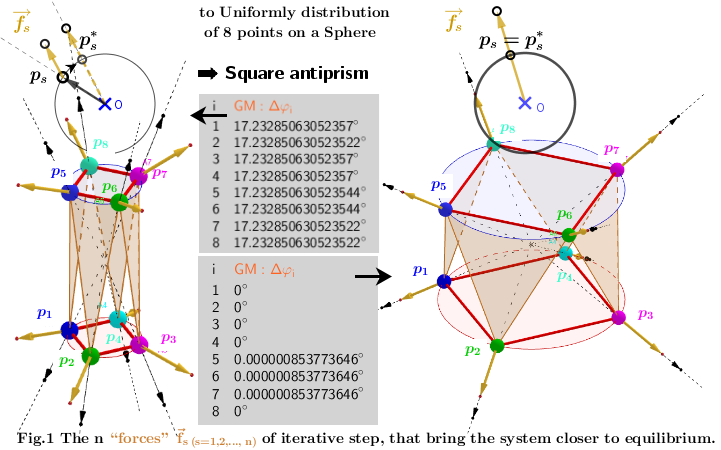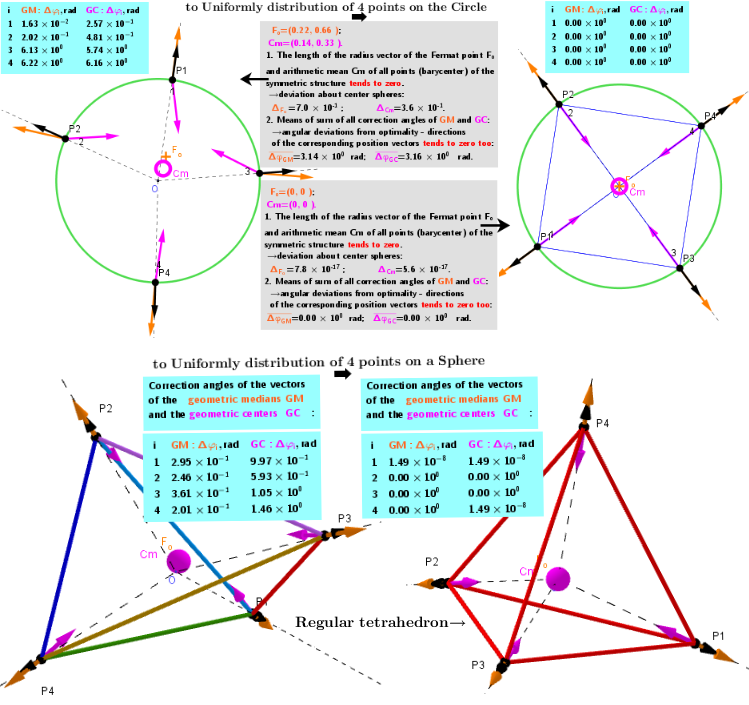# Method of Lagrange multipliers. Placing points on a circle/sphere such that the each point is the geometric median of all its other points.

Main Problem: to find the algorithm of finding a uniform distribution of points on a sphere. Search method: to find critical points of "specific" functional dependencies and understand "by simple models " to which particle distributions this leads. The main idea of this applet - to find on the circle/sphere such location of points, that each of them is at the point of the geometric median of its other points, i.e. to maximize the sum of the mutual distances of all particles: Applet. Method of Lagrange multipliers. Relative positioning of "repulsive" movable points on a circle Applet. Finding the optimal relative position of the" repulsive " set of particles on the circle Applet. Finding the optimal relative position of the" repulsive " set of particles on the sphere Applet Some cases of polyhedra with an extreme distribution of their vertices on the surface of a sphere. Applet. Polyhedra whose vertices are equivalent and have an extreme distribution on the same sphere. Applet. Example of non-uniqueness of the extreme distribution of n=16 particles on the surface of a sphere. Applet. 3d shapes: n=72. Extreme distribution of points on the surface of a sphere and comparison with two known but not extreme ones having the same number of vertices Images. Placing points on a sphere such that the each point is the geometric median of all its other points. The Geometric Median(GM) of a discrete set of sample points in a Euclidean space is the point minimizing the sum of distances to the sample points or Fermat point Fo. Let us generalize this definition for a set of "repulsive" points on the surface of a circle/sphere. Obviously, here is relevant the point on a circle/sphere, maximizing the sum of distance (Distance Sum) between every possible pair of points on a circle/sphere. It is of interest to find out such mutual arrangement of repulsive set of particles on a sphere, when each point of this set is Geometric Median(GM) of the remaining n-1 points. We assume that the equilibrium - stationary state in system of charges is reached if the sum of their mutual distances is maximal. Iterative approach of particle placement is applied for achieving a stationary state. Problem statement: to place n points on the circle/sphere so that each point is located at a position of geometric median of remaining n-1 others points. And it is necessary to take into account the restriction: each this point must remain on the original circle/sphere. The problem is solved by the method of Lagrange multipliers to maximize the the function of sum of distances (Distance Sum). The proposed iterative procedure is applied sequentially to each point until the correction vectors will not be parallel to their corresponding positional vectors: the gradient of the Distance Sum is perpendicular to the constraint-circle/sphere. In the following, we will consider the case of distribution of points on the sphere. Spherical Distribution of n Points with Maximal Distance Sum Solution method: There is a set lP={p1, p2,...,pn} of points on a sphere and {(xi,yi,zi)∈S:i= 1,...,n} -their coordinates. Let S be a sphere of radius R centered at the origin (O): S:={||||∈ℝ³: ||||=R}. The Geometric Median is defined here as point on a sphere from where the function of sum of the distances to each point pi's have at that point: local minimums, maximums or saddle points. Critical points can be found using Lagrange multipliersas (Λ(x,y,z,λ)=f(x,y,z)+λ*g(x,y,z)) finding the Extreme values of the function : f(x,y,z)= -sum of the distances from (x,y,z) to each point pi's, subject to a constraining equation: g(x,y,z)= - point (x,y,z)∈S. I.e. it is necessary to find the critical points f (x,y,z) subject to: g (x,y,z)=0. Further we will use positional vectors :=(x,y,z)T : f(x,y,z)=and g(x,y,z)=-R. The gradients are: ∇f∼and ∇g =/R -gradient of the magnitude of a position vector r. There is a system of equations: ∇f(x,y,z)= λ*∇g(x,y,z). A local optimum occurs when ∇f(x,y,z) and ∇g(x,y,z) are parallel, and so ∇f is some multiple of ∇g.There are no explicit Geometric Medians formulas, in contrast to Geometric Centers explicit formulas. In our case, the solution of the system of equations can be found use iterative procedures. I propose iterative procedures in which each step produces is a more accurate approximation. Relationship between vectors of two consecutive iterations is defined by finds the Maxima - points. That means that the := (sum of unit vectors location of a point ps relative to others n-1 points) -is the collective "force" action at point ps (as shown on the Fig. 1 above . All points are successively corrected until the system of points comes to a stationary state. Finally, at the end of iterations they must match ps✱=ps for all s=1,2,...,n and vectors fs will be perpendicular to the surface S of the sphere at all points from lP. The iteration is terminated when the center of mass of n particles on a sphere (with a certain degree of accuracy) will not be in the center of the sphere). Spherical Distribution of n Points with Maximal quadrat Distance Sum Solution method: The Geometric Center is defined here as point on sphere from where the sum of the squares of all Euclidean distances to each point pi's have at that point: local minimum, maximum or a saddle points. Critical points can be found using Lagrange multipliersas (Λ(x,y,z,λ)=f(x,y,z)+λ*g(x,y,z)) finding the Extreme values of the function : fq(x,y,z)= -sum of the squares of the distances to the points pi, subject to a constraining equation: g(x,y,z)=x2+y2+z2-R2 - points (x,y,z)∈S. I.e. it is necessary to find the critical points fq(x,y,z) subject to: g(x,y,z)=0. Let's denote the resulting point as Sum(lP):= , then ∇f/2=n*(x,y,z)- or ∇f/2=n*(x,y,z)-Sum(lP) and ∇g/2=(x,y,z): ⇒(x,y,z)∼Sum(lP). The point (x,y,z) that minimize the sum of the squares of the distances to the points pi is the Geometric Center (gravity center, barycenter, center of mass, centroid) GC: Cm= -its coordinates are the averages of the coordinates of the points from set lP. In our case, the position of the points on a sphere must be defined as: ✱=R*UnitVector( ) and :=. However, this iteration scheme does not converge. Iterations in this case do not lead to stationary positions of the particles. Notation in applets: position vector(s)∼lP(s) -position vector for each point s; GM vector(s) ∼Sum[Zip(UnitVector(lP(s)-a),a,lP\lP(s))] - the vector of the geometric median(GM) at point ps caused by the „action“ of the remaining (n-1) points; GC vector(s) ∼Sum[lP\lP(i)]/(n-1) - the vector of the geometric center(GC) at point ps caused by the „action“ of the remaining (n-1) points; Δφs:=Angle(position vector(s),GM vector(s)); Δφs:=Angle(position vector(i),GC vector(s)).Results. In the applet in the tables, you can see the angular distances Δφi between position vectors and corresponding vectors of geometric medians and centers for each particle. In the equilibrium state (as it is demonstrated by calculations), the positions of the points on the sphere are both geometric medians and geometric centers of other points on the sphere. Correction angles are Angle measurement between the corresponding Black and Orange Vectores. The condition Δφi → 0 is an equilibrium condition for “repulsive” points on the surface of the sphere: there are no “tangential forces” that will move them on the surface of the sphere. The mean of the sum of all correction angles Geometric medians-GM and Geometric centers-GC Δφs tend to zero too. Denote these values respectively as ΔφGM and ΔφGC . For symmetric structures, the length of the position vectors of the Fermat point Fo andcentroid Cm tends to zero. Let us denote by ΔCm and ΔF_o -the deviations of these values with respect to the center of the sphere.
Explanation. In the case of points on a sphere: stop animation is performed by pressing the button-Stop or automatically, provided that the geometric center of the points coincides (with a certain degree of accuracy) with the center of the sphere: ΔCm<2*10-k. In the upper left part of the applet there are in two columns two (for convenience of comparison) subsequent iterations of the Distance Sum. Their values are calculated as the average distance between particles on the Unit Sphere : Distance Sum/[0.5*n*(n-1)*R]. The table of values of these values for different values n are given in https://www.geogebra.org/m/qmguscwp.